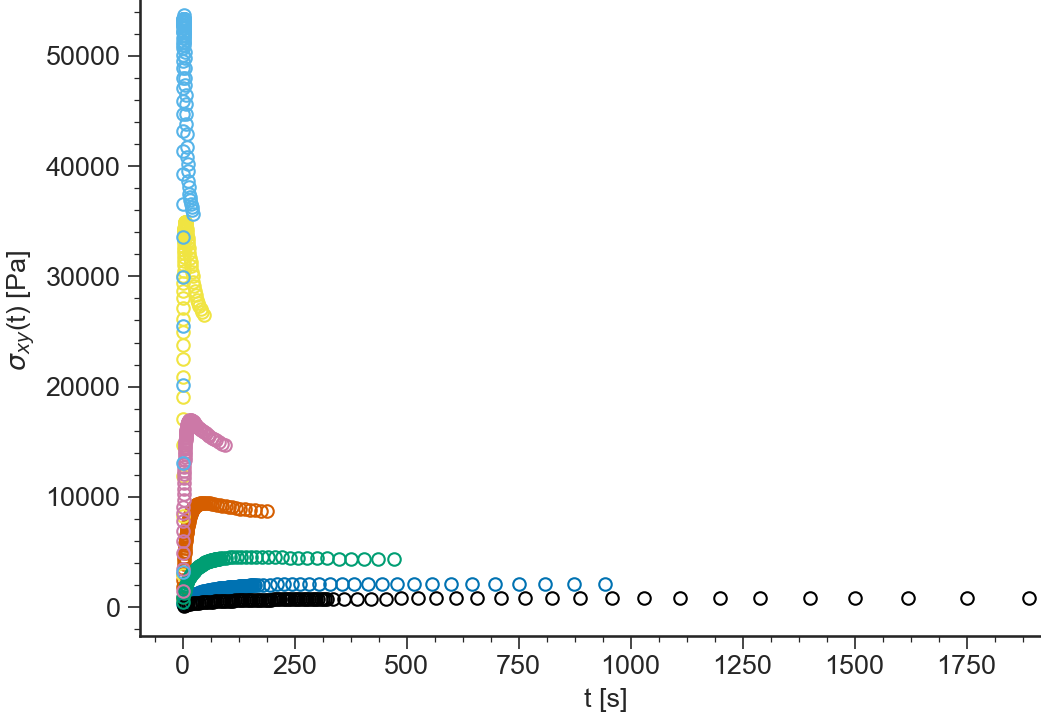# NLVE: General description¶

## Purpose¶

Application to Analyze Start up of Nonlinear flow

## Data Files¶

• The first line of the file should contain the sample parameters separated by semi-colons (;). It may contain any number of parameters which will be read and saved as file-parameter in RepTate.

• Then the data columns should appear, separated by spaces or tabs.

### .shear extension¶

Text files with .shear extension should be organised as follows:

• .shear files should provide parameter values for

1. shear rate, $$\dot\gamma$$

2. temperature, $$T$$

• 2 columns separated by spaces or tabs containing respectively:

1. time, $$t$$,

2. shear stress growth function, $$\sigma^+(t)$$,

A correct .shear file looks like:

gdot=0.1;T=160;
2.50E-01 845
7.50E-01 1830
1.25E+00 2490
...      ...


### .uext extension¶

Text files with .uext extension should be organised as follows:

• .uext files should provide parameter values for

1. Hencky strain rate, $$\dot\varepsilon$$

2. temperature, $$T$$

• 2 columns separated by spaces or tabs containing respectively:

1. time, $$t$$,

2. tensile stress growth function, $$\sigma_E^+(t)$$,

A correct .uext file looks like:

gdot=0.1;T=160;
2.50E-01 845
7.50E-01 1830
1.25E+00 2490
...      ...


Warning

The first line of the .uext file provides the flow rate as gdot=0.1, not edot=0.1

## Views¶

### log(eta(t))¶

BaseApplicationNLVE.viewLogeta()[source]

Logarithm of the transient shear or extensional viscosity (depending on the experiment) $$\eta(t)$$ vs logarithm of time $$t$$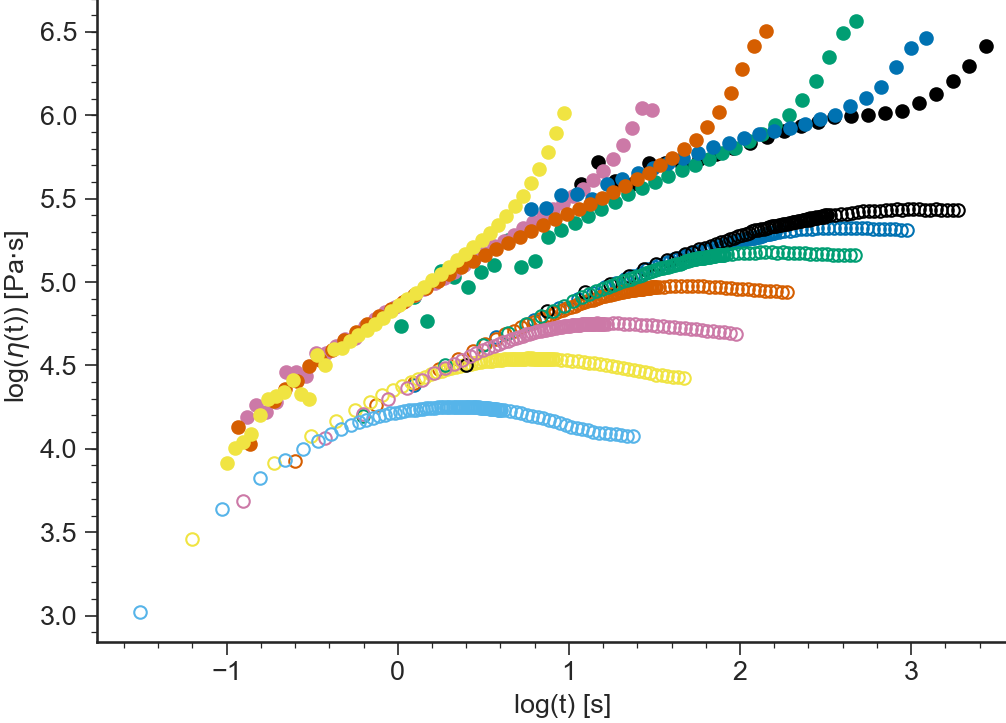### eta(t)¶

BaseApplicationNLVE.vieweta()[source]

Transient shear or extensional viscosity (depending on the experiment) $$\eta(t)$$ vs time $$t$$ (both axes in logarithmic scale by default)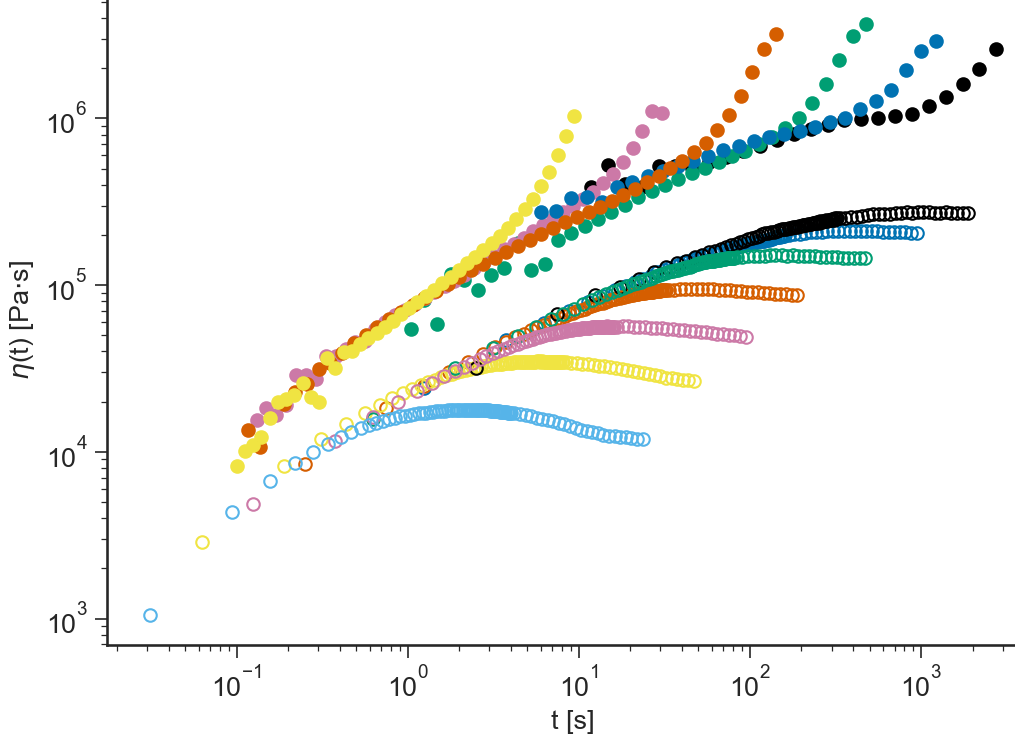### log(sigma(gamma))¶

BaseApplicationNLVE.viewLogSigmaGamma()[source]

Logarithm of the transient shear or extensional stress (depending on the experiment) $$\sigma(t)$$ vs logarithm of the strain $$\gamma$$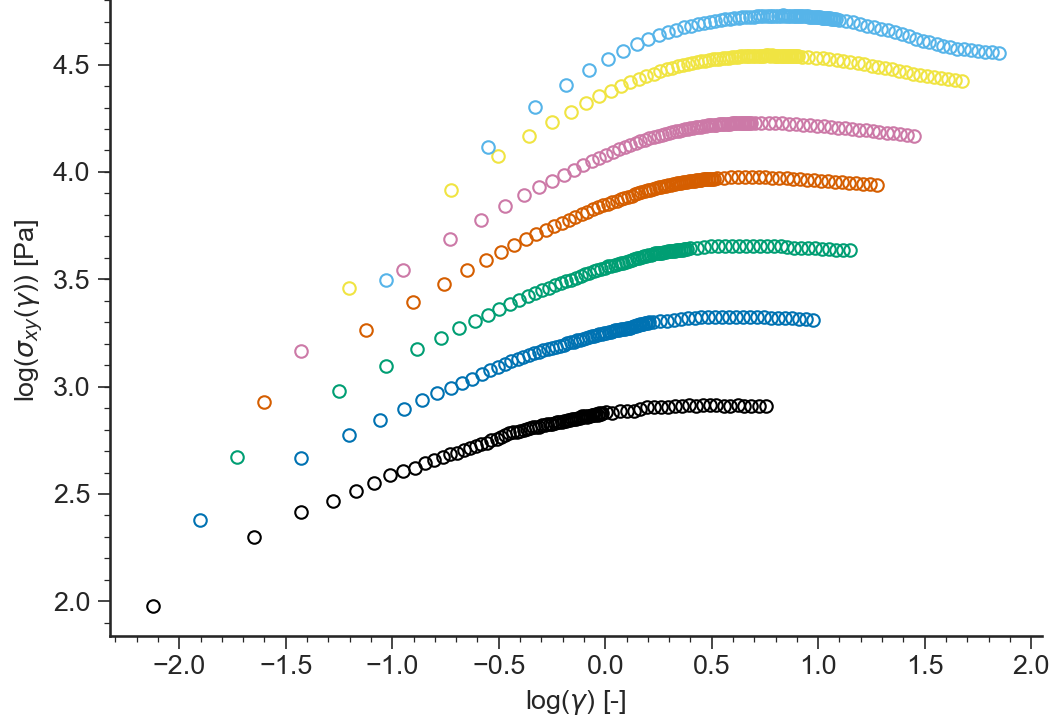### sigma(gamma)¶

BaseApplicationNLVE.viewSigmaGamma()[source]

Transient shear or extensional stress (depending on the experiment) $$\sigma(t)$$ vs strain $$\gamma$$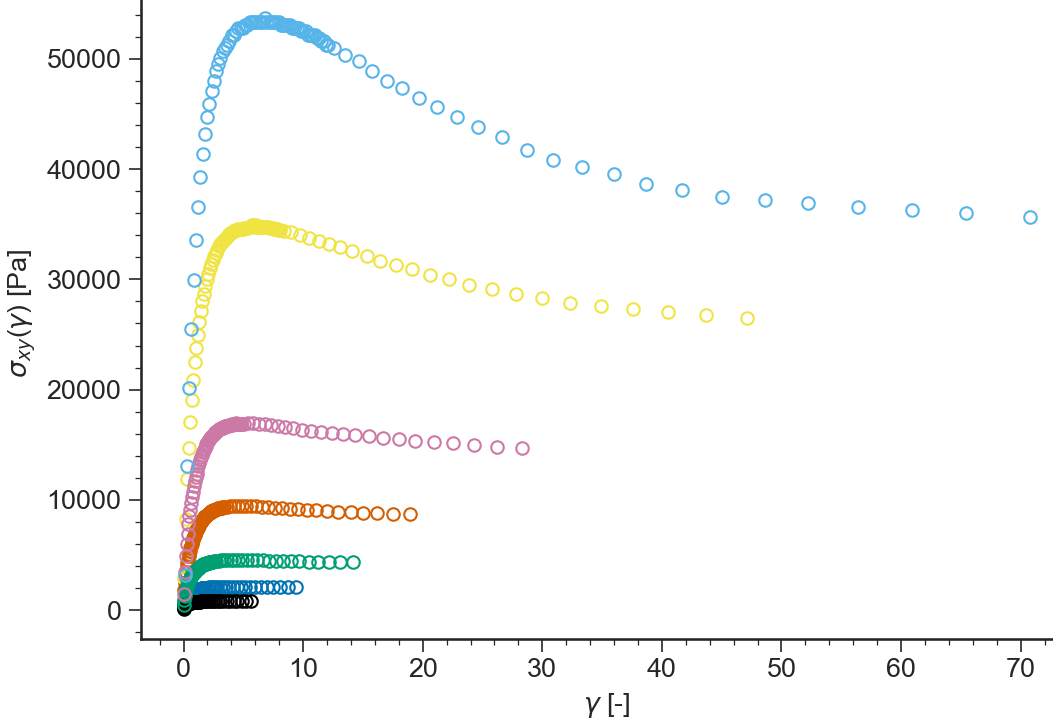### log(sigma(t))¶

BaseApplicationNLVE.viewLogSigmaTime()[source]

Logarithm of the transient shear or extensional stress (depending on the experiment) $$\sigma(t)$$ vs logarithm of time $$t$$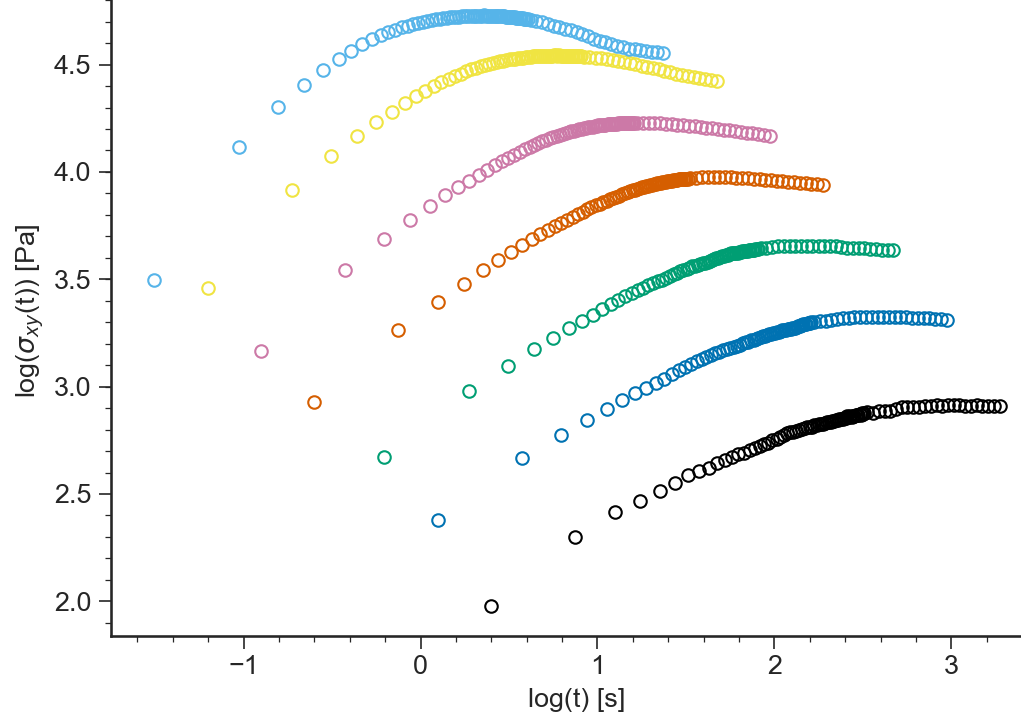### sigma(t)¶

BaseApplicationNLVE.viewSigmaTime()[source]

Transient shear or extensional stress (depending on the experiment) $$\sigma(t)$$ vs time $$t$$